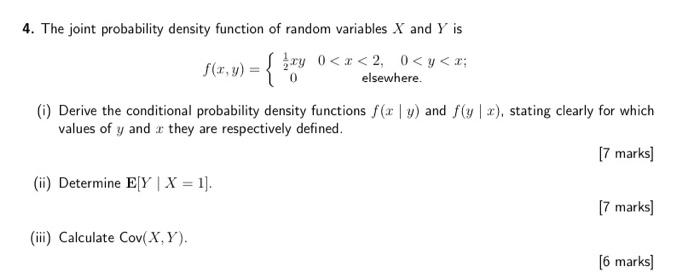# (Solved): can you solve q4 please? 4. The joint probability density function of random variables $$X$$ and \ ...4. The joint probability density function of random variables $$X$$ and $$Y$$ is \[ f(x, y)=\left\{\begin{array}{cc} \frac{1}{2} x y & 0

We have an Answer from Expert